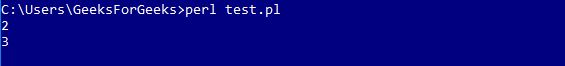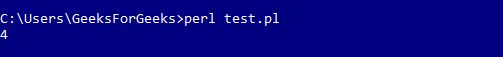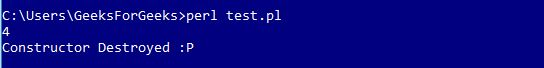GFG App
Open AppBrowser
Continue

# Perl | Constructors and Destructors

Constructors

Constructors in Perl subroutines returns an object which is an instance of the class. In Perl, the convention is to name the constructor “new”. Unlike many other OOPs, Perl does not provide any special syntax for constructing an object. It uses Data structures(hashes, arrays, scalars) that have been explicitly associated with that particular class.
The constructor uses the “bless” function on a hash reference and the class name (the name of the package).

Let’s design some codes for better explanations:
Note: The following codes will not Run on Online IDE because of the use of Packages. The code below is a Perl class or a module file. Save the below file as (*.pm) extension.

 `# Declaring the Package ` `package` `Area;  ` ` `  `# Declaring the Constructor method ` `sub` `new  ` `{ ` `    ``return` `bless` `{}, ``shift``; ``# blessing on the hashed  ` `                            ``# reference (which is empty). ` `} ` ` `  `1; `

When the constructor method is called, the package name ‘Area’ is stored in the default array “@_”. The “shift” keyword is used to take the package name from “@_” and pass it on to the “bless” function.

 `package` `Area; ` ` `  `sub` `new ` `{ ` `    ``my` `\$class` `= ``shift``; ``# defining shift in \$myclass ` `    ``my` `\$self` `= {}; ``# the hashed reference ` `    ``return` `bless` `\$self``, ``\$class``; ` `} ` `1; `

`Note: "my" restricts the scope of a variable.`

Attributes in Perl are stored as the key, value pairs in the hashed reference. Further, adding some attributes to the code.

 `package` `Area; ` ` `  `sub` `new  ` `{ ` `    ``my` `\$class` `= ``shift``; ` `    ``my` `\$self` `=  ` `    ``{ ` `        ``length` `=> 2, ``# storing length ` `        ``width`  `=> 3, ``# storing width  ` `    ``}; ` `    ``return` `bless` `\$self``, ``\$class``; ` `} ` `1; `

The above code(the Area Class) has the two attributes : length and width. To get an access to these attributes, another Perl program is designed to use them.

 `use` `strict; ` `use` `warnings; ` `use` `Area; ` `use` `feature ``qw/say/``; ` ` `  `# creating a new Area object ` `my` `\$area` `= Area->new; ` ` `  `say` `\$area``->{``length``}; ``#print the length ` `say` `\$area``->{width}; ``# print the width `

Steps to Run the Code:

1. Save the Program with Package Area in a text file named as Area.pm
Note: Name of the file should always be same as the name of the package.
2. Now, save the program that is used to get access to the attributes defined in the package by the name *.pl. Here, * can be any name(In this case it is test.pl).
3. Run the code saved as test.pl in the Perl command line by using the command
perl test.pl

Output:Passing Dynamic attributes :
Update the existing files with Dynamic attributes:

Areas.pm:

 `package` `Area; ` ` `  `sub` `new  ` `{ ` `    ``my` `(``\$class``, ``\$args``) = ``@_``; ``# since the values will be  ` `                             ``# passed dynamically ` `    ``my` `\$self` `=  ` `    ``{ ` `        ``length` `=> ``\$args``->{``length``} || 1, ``# by default the value is 1 (stored) ` `        ``width`  `=> ``\$args``->{width} || 1,  ``# by default the value is 1 (stored) ` `    ``}; ` `    ``return` `bless` `\$self``, ``\$class``; ` `} ` ` `  `# we have added the get_area function to ` `# calculate the area as well ` `sub` `get_area  ` `{ ` `    ``my` `\$self` `= ``shift``; ` `     `  `    ``# getting the area by multiplication ` `    ``my` `\$area` `= ``\$self``->{``length``} * ``\$self``->{width};  ` `    ``return` `\$area``; ` `} ` `1; `

test.pl:

 `use` `strict; ` `use` `warnings; ` `use` `feature ``qw/say/``; ` `use` `Area; ` ` `  `# pass length and width arguments  ` `# to the constructor ` `my` `\$area` `= Area->new( ` `            ``{ ` `                ``length` `=> 2, ``# passing '2' as param of length ` `                ``width` `=> 2, ``# passing '2' as param of width ` `            ``}); ` ` `  `say` `\$area``->get_area; `

Now, the Params

`length = 2, width = 2`

is passed onto the package Area, to calculate the area of the Square.

The arguments are contained in the default array variable @_ when any subroutine is called

Note: Now follow the same process of running the code as explained above.
Output:Destructors

Perl automatically calls the destructor method when all the references to an object go out of scope. Destructor methods are of use if the class creates threads or temporary files that need to be cleaned up if the object gets destroyed. Perl contains a special method name, ‘DESTROY’ for the destructor, that must be used at the time of destructor declaration.

Syntax:

 `sub` `DESTROY  ` `{  ` `    ``# DEFINE Destructors ` `    ``my` `\$self` `= ``shift``; ` `    ``print` `"Constructor Destroyed :P"``;  ` `} `

`Once this snippet is added to existing file Area.pm.`

The output will be somewhat like this:My Personal Notes arrow_drop_up